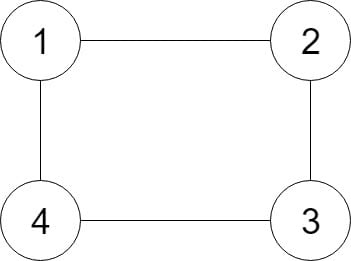# 133. Clone Graph

Reference: LeetCode
Difficulty: Medium

## Problem

Given a reference of a node in a connected undirected graph, return a deep copy (clone) of the graph. Each node in the graph contains a val (int) and a list (List[Node]) of its neighbors.Note:

• The number of nodes will be between 1 and 100.
• The undirected graph is a simple graph, which means no repeated edges and no self-loops in the graph.
• Since the graph is undirected, if node p has node q as neighbor, then node q must have node p as neighbor too.
• You must return the copy of the given node as a reference to the cloned graph.

## Analysis

### DFS (recursion)

Optimization: We can combine dfsSetMap and dfsSetNeighbors to get clean code!

Time: $O(V + E)$
Space: $O(V)$

CommentJunhao Wang
a software engineering cat